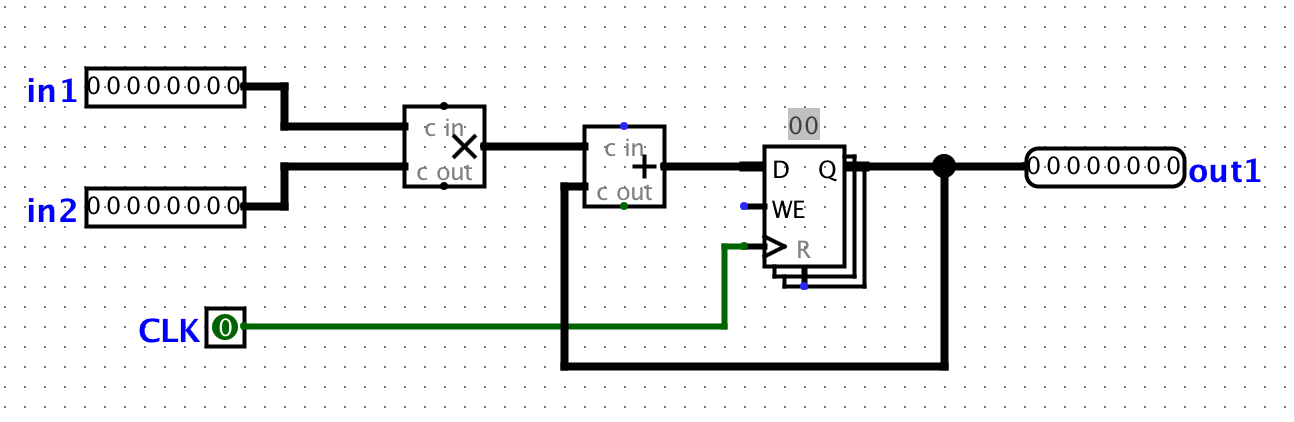## Lab 7

Computer Architecture I ShanghaiTech University
Lab 6 Lab 7 Lab 8

## Exercises

### Exercise 1: Fibonacci Numbers

So far we have built circuits that are either 1) purely combinational and require no clock or 2) have memory but run infinitely. In this exercise, we want to build a circuit that will compute the Nth Fibonacci number. As a quick review the Fibonacci numbers are defined by F0 = 0, F1 = 1, and Fi = Fi-1 + Fi-2.

1. Start with just the infinite Fibonacci computation. Hopefully this should be pretty intuitive based on previous exercises that you have done before. How many registers do you need? What arithmetic blocks do you need? Where should the output be attached? Make sure you figure out a way to properly initialize the registers for the computation to work.
2. Your sub-circuit will compute up to the 15th Fibonacci number and we will assume our input N > 0 is an unsigned number. How many input bits do you need and how many output bits do you need to represent the 15th Fibonacci number?
3. Now to prevent the circuit from computing the Fibonacci numbers to infinity, we will make use of the Enable bit (lower left) on registers. When unset or pulled high, the registers will continue to load their inputs on the rising edge of the CLK. If Enabled is pulled low, then the registers will NOT load their inputs. We need to create a signal that will properly go low when we want the circuit to stop computing Fibonacci numbers.
4. Create an additional part of the circuit that halts the original circuit after N computations. For this exercise you may find the following blocks useful: Counter (Memory), Comparator (Arithmetic), and Probe (Wiring). Make sure that it properly stops on the Nth Fibonacci number (watch for off-by-one errors) and that it actually stays there (run it for at least 20 clock cycles).
Your output should be in binary, but you can use a probe to display the value in decimal. Make sure you check the attributes of any counter and comparator you use.

Bonus: With proper register initialization, you can get this circuit fib15 to work properly for N > 0. But register initialization is annoying and must be repeated every time you reset your circuit. With a clever addition, you can get this fib15 to work for N >= 0 without the need for register initialization (Hint: it involves a MUX).

#### Checkoff

• Show your TA your fib15 circuit and verify that for N = 15 after some time it will return Out = 610 indefinitely.

### Exercise 2: Inefficiencies Everywhere

For this exercise, we can assume that registers initially carry the value zero. We will be using the lab file `exercise1.circ`, which should have a subcircuit called `non_pipelined` which looks something like this:All this circuit does is take in two inputs, multiply them together, then adding it to the current state value. For this circuit, let the propagation delay of an adder block be 50ns, the propagation delay of a multiplication block be 55ns, and the CLK-to-Q delay of a register is 5ns. Assuming no setup time and hold time, calculate the maximum clock rate at which this circuit can operate. Assume that both inputs come from clocked registers that receive their data from an outside source.

#### Checkoff

• Show your TA the calculations you performed to find the maximum clock rate (non-pipelined).

### Exercise 3: Pipe that Line

We want to improve the performance of this circuit and let it operate at a higher clock rate. In order to accomplish this, we want to have two stages in our pipeline: a multiplication stage and an addition stage, in that order.

In order to check that your pipelining still produces correct inputs, we will consider the outputs from the circuit “correct” if and only if it corresponds to the sequence of outputs the non-pipelined version would emit, bar some leadings zeroes. For example, if for some sequence of inputs, the non-pipelined version emits the sequence [3, 5, 1, 2, 4, …]. Then, the correct pipelined circuit might emit the sequence [0, 3, 5, 1, 2, 4, …] for the same sequence of inputs.

In your `exercise1.circ` file, the `main` circuit is set up to produce the output sequence [3, 5, 1, 2, 4, -1, 0, 0, …] from the non-pipelined version of the circuit. The ROM blocks should be initialized to the proper inputs, but if something goes wrong, select the ROM, click on “Contents”, click “Open”, then choose `Romdata`.

Note that in order to pipeline the circuit, we need a register to hold the intermediate value of the computation between pipeline stages. This is a general theme with pipelines.

• Complete the sub-circuit `pipelined`. You will need to add a register to divide the multiplication and addition stages up.